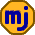## Tuesday, February 26, 2013

### Adding Commodities to a Portfolio

Larry Swedroe has long advocated adding a small amount of commodities to a portfolio to boost risk-adjusted returns. The theory says that while the commodities lower the overall expected return, they more than make up for this with their lowering of the portfolio risk (standard deviation). I’ve been resistant to this idea despite Swedroe’s numerical examples of improved risk-adjusted return. I think I can finally explain my reluctance when it comes to commodities.

In Swedroe’s most recent book (see my review here), he gives a clear example showing the effect of adding some commodities to a particular portfolio based on historical returns from 1975-2011. The portfolio begins with a 60/40 split between various equities and 5-year treasury notes, and has the following characteristics.

Before Commodities

Annualized Return: 12.4%
Annual Standard Deviation: 11.8%

He then replaces part of the equities to give the portfolio a 4% exposure to commodities.

After Commodities

Annualized Return: 12.1%
Annual Standard Deviation: 11.2%

So, the question is whether it is worth it to reduce expected returns by 0.3% to get a 0.6% reduction in volatility. Let’s first look at this in terms of relative risk aversion (RRA). A rough calculation of RRA is the change in expected return divided by the change in half the square of the standard deviation. Half the square of standard deviation dropped by

(1/2)(11.8% x 11.8% - 11.2% x 11.2%) = 0.069%

The expected return dropped by 0.3% which gives RRA = 0.3/0.069 = 4.3. In fact, the real figure is closer to 5.3 because I think Swedroe gave compounded returns rather than arithmetic average returns. This is an extremely high level of risk aversion, well beyond my own fear of risk.

On the other hand, if we look at the numbers another way, the commodities make sense. Let’s project this risk reduction to see what would happen if we were able to extend it further. If we repeatedly reduce expected return by 0.3% and volatility by 0.6%, we get to a risk-free return at 6.5%. This is clearly higher than the real risk-free return. So, mixing in some commodities is more efficient at reducing volatility than mixing in risk-free investments.

This means that if we want lower volatility than we would get from an all-equities portfolio, then it makes sense to add some commodities first, and then add in some risk-free (or very low-risk) investments.

This is great for most investors, but I don’t seek lower volatility. I’m happy to take on volatility as long as it comes with sufficiently higher expected return. So, I don’t want government debt for my long-term savings, and I don’t want commodities either.

A counterargument to this reasoning that Modern Portfolio Theory would spit out is that I should mix in commodities and then leverage my portfolio a little. The theory says this approach would give me a slightly higher expected return than an all-stock portfolio with the same volatility.

The problem with this result is that it is based on the baked-in assumption that I can borrow at the risk-free rate. I can’t. Borrowing always costs me more than I can get from a risk-free investment. If I back the loan against my house, I can make the difference between my borrowing rate and the risk-free rate quite small, but this gap is still enough to kill the idea of using commodities. So, my portfolio will remain commodity-free.

1.1.